# Expanding in (sine, cosine) Fourier series: Methods survey

Question: Find the Fourier series for a function f (t) that is either T-periodic or given on some interval of the form [a,a + T ). Find the sum of this series.

Solution: First determine the frequency ω = 2π/T and then find coefficients by evaluating integrals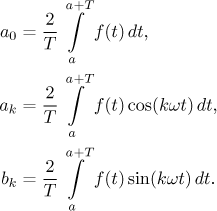The Fourier series is then obtained by writing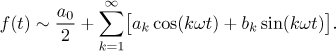If f is nice enough (see Jordan's conditions), you can determine the sum of the resulting Fourier series. First, draw the T-periodic function f (either it was given as such or it was given on a suitable interval, then draw its periodic extension). Then, at every point of discontinuity, draw a dot at the level that is the average of the left and right value there. This is the sum of the Fourier series.

Note: The coefficient a0 is actually given by the same formula as the other ak, so we could just specify one formula. However, for k = 0 the cosine becomes 1 and in practice we therefore evaluate a0 separately from the other integrals anyway. Thus it is convenient to put it aside as a special case.

Question: Find the sine Fourier series for a function f (t) that is given on some interval of the form [0,L ). Find the sum of this series.

Solution: The sine series will be based on an odd extension with period T = 2L. First determine the frequency ω = 2π/T = π/L. Then find coefficients bk as above but with L in place of T, set a0 and ak equal to zero and write the series exactly as above.

If f is nice enough (see Jordan's conditions), you can determine the sum of the resulting Fourier series. First, draw the given function. Second, extend the graph also to the interval (−L,0) in such a way that the resulting function is odd (flip the given graph about both axes). Then draw the T = 2L-periodic extension of this odd function and handle points of discontinuity exactly as above.

Question: Find the cosine Fourier series for a function f (t) that is given on some interval of the form [0,L ). Find the sum of this series.

Solution: The cosine series will be based on an odd extension with period T = 2L. First determine the frequency ω = 2π/T = π/L. Then find coefficients a0 and ak as above but with L in place of T, set bk equal to zero and write the series exactly as above.

If f is nice enough (see Jordan's conditions), you can determine the sum of the resulting Fourier series. First, draw the given function. Second, extend the graph also to the interval (−L,0) in such a way that the resulting function is even (flip the given graph about the y-axis). Then draw the T = 2L-periodic extension of this even function and handle points of discontinuity exactly as above.

It is often possible to improve the resulting series by using these handy formulas.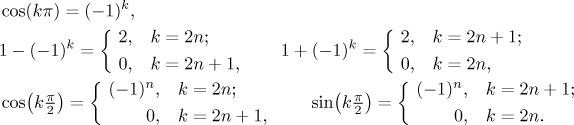Example: Find the Fourier series, the sine Fourier series and the cosine Fourier series for the function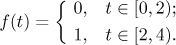This function can be extended to a function with period T = 4, thus the frequency is ω = π/2. We find the coefficients: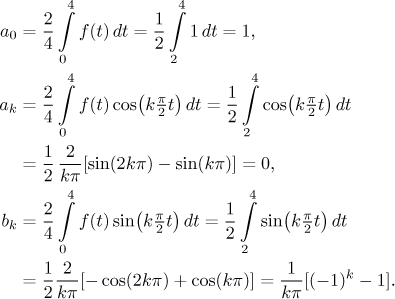Thus we get the series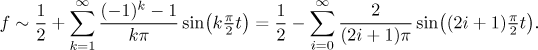We noticed that in the series, all even coefficients are zero and odd ones are −2, so we rewrote it accordingly.

The sine and cosine series have L = 4, T = 8, and ω = π/4. The sine series has ak = 0 and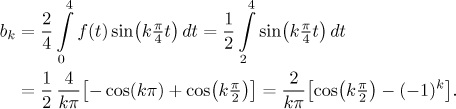Thus the sine Fourier series is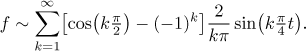The cosine Fourier series has bk = 0 and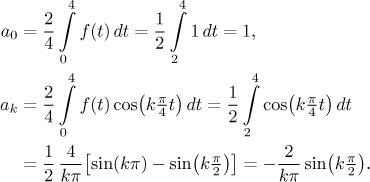Thus the cosine Fourier series is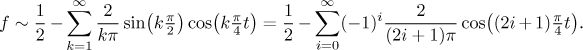Now we determine the sums of the above three series. In the following picture there are six graphs. The first is the periodic extension of the given function and the second is the sum of the Fourier series by Jordan's criterion. The third graph is the odd periodic extension and then there is the sum of the sine Fourier series. Finally, the fifth graph is the even periodic extension and then the sum of the cosine Fourier series.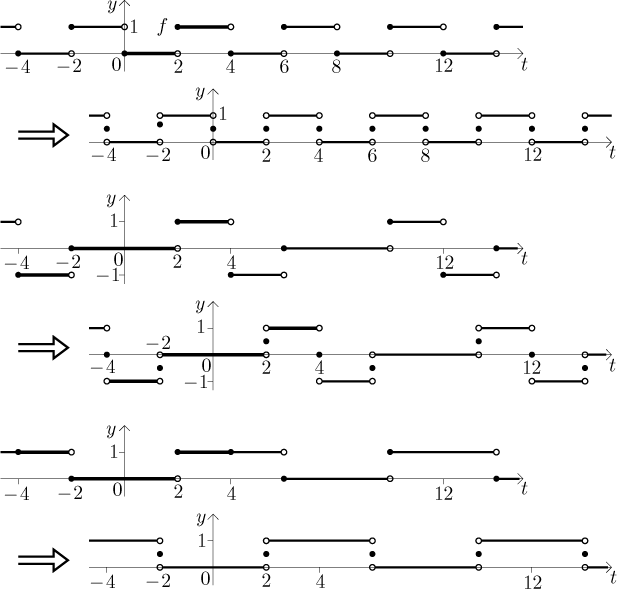Note that the Fourier series has the form of 1/2 plus a sine series. Indeed, if we take the periodic extension of f and move it down by 1/2, we get an odd function whose Fourier series necessarily features only sines (and thus it is also its sine Fourier series).